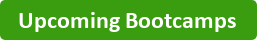Do you already have SQL knowledge? Need to spruce up your skillset with calculations and extracting data from multiple tables ? Then, this is the SQL Bootcamp for you. The Intermediate SQL Bootcamp is a 3 course Bootcamp that takes place over 3 consecutive Saturdays (Bootcamp price includes all 3 courses). The courses will cover the following:Course I: Calculating Intermediate Mathematical Operations and Filtering Aggregated Data

• Perform mathematical operations on multiple fields
• Calculate formulas using mathematical operations and aggregate function
• Perform filtering using HAVING vs. WHERE statements
• Use HAVING vs. WHERE statement with mathematical formulas and calculations

Course II: Retrieving Data from Multiple Tables

• Retrieve data from at least 4 tables using Joins